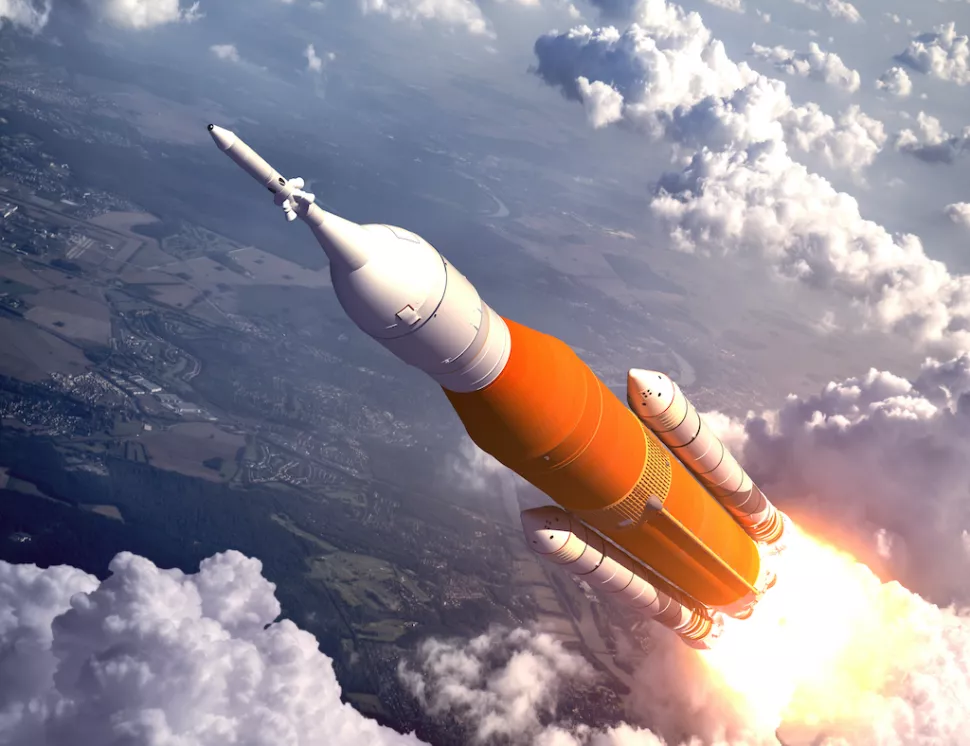Sarah Taylor

HS-PS2-1

# Average Acceleration Study Guide

Acceleration is much more complex than the everyday concept of speeding up as we mostly know it to be!

# INTRODUCTION

Rockets are strong machines that, by hurling us out of orbit with their enormous exhaust velocities, allow us to see and study space. The high exhaust velocity must accelerate the shuttle from zero to 8,000 meters per second (nearly 18,000 miles per hour) in eight minutes to achieve the threshold height required to orbit the Earth, which means that the rocket will go up by 16.6 minutes every second! Now the question that arises is, what is acceleration?Source

## ACCELERATION

• Understanding acceleration’s meaning is important in our everyday lives, as well as in the wide expanses of space and the microscopic world of subatomic physics.

• Accelerate is a verb that signifies "to speed up" in ordinary language, but in the language of physics, the rate of change of velocity is known as acceleration.

• It is symbolized by the letter 'a', and the SI unit of acceleration is m/s2.

• The formula for acceleration is a = dv/ dt, where dv is the change in velocity and t is the time elapsed.

• The acceleration can be positive, negative, or zero depending upon the velocity and its direction.

• Acceleration in the line of velocity is referred to as positive acceleration.

• Negative acceleration occurs when velocity is increased in the reverse direction.

The term "zero acceleration" refers to a body that is not accelerating; in this situation, the velocity of the body is constant.

## TYPES OF ACCELERATION

Mainly uniform acceleration, non-uniform acceleration, and average acceleration are the three types of accelerated motions.

• Uniform acceleration: An object in uniform accelerated motion travels the same distance in the same amount of time, regardless of the length of time.

• Non-uniform acceleration: Regardless of the time period length, a non-uniform accelerated motion item does not travel the very same distance in equal intervals of time.

## AVERAGE ACCELERATION

The average acceleration during a certain interval is accompanied by a change in velocity for that period. The average acceleration, unlike acceleration, is measured for a certain interval. For a given interval of time, the average acceleration is denoted as

Av ( average acceleration ) = v-v0 / t2- t1 ,

where v0 and v are the instantaneous velocities at time t2 and t1.

# SUMMARY

• The rate of change of velocity is known as acceleration.
• Uniform acceleration, non-uniform acceleration, and average acceleration are the three types of accelerated motions.
• Average acceleration is the change in the velocity of an object for a particular period.
• The average acceleration is denoted as

Av ( average acceleration ) = v-v0 / t2- t1 , where v0 and v are the instantaneous velocities at time t2 and t1.

## FAQs

1. What is the difference between Acceleration and Velocity?

The velocity of the object is defined as the quantity of motion normalized to time, whereas Acceleration is a vector quantity that describes how quickly an item's velocity changes.

2. What is centripetal acceleration?

The acceleration of an object traveling in a circular route is known as centripetal acceleration.

3. What is tangential acceleration?

Tangential acceleration is measured as the rate at which the tangential velocity of an object in a circular motion changes in magnitude.